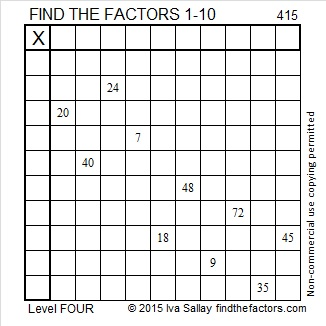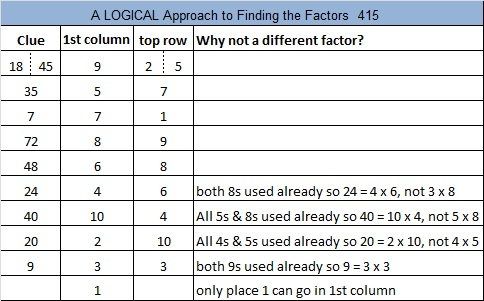# 415 and Level 4

415 is obviously a multiple of 5 so it is a composite number. Scroll down past the puzzle to view its other factors.Print the puzzles or type the factors on this excel file: 10 Factors 2015-03-02

• 415 is a composite number.
• Prime factorization: 415 = 5 x 83
• The exponents in the prime factorization are 1 and 1. Adding one to each and multiplying we get (1 + 1)(1 + 1) = 2 x 2 = 4. Therefore 415 has exactly 4 factors.
• Factors of 415: 1, 5, 83, 415
• Factor pairs: 415 = 1 x 415 or 5 x 83
• 415 has no square factors that allow its square root to be simplified. √415 ≈ 20.3715## 2 thoughts on “415 and Level 4”

1.abyssbrain

It’s also interesting to note that 415 is the sum of five consecutive squares

7² + 8² + 9² + 10² + 11² = 415

•ivasallay

Thank you for the additional information!

This site uses Akismet to reduce spam. Learn how your comment data is processed.